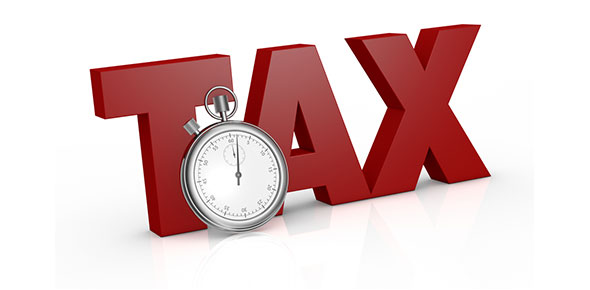# Tax, Tip, Discount, I=prt, Percent Of Change

20 Questions | Attempts: 789
ShareSettingsThis is a quiz that asks different random questions that are designed to test your knowledge on tax, tip discount and percentage of change. It might not be as easy as you think but just try it out to see how you it goes.

• 1.
Mrs. York's homeroom recorded the total number of books read per month.  In May, they read 72 books, and in June they read 56 books.  What was the approximate percent decrease from May to June?
• A.

4% decrease

• B.

4.5% decrease

• C.

16% decrease

• D.

22% decrease

• 2.
The cost of a lamp is \$29.95.  Sales tax is 6%.  About how much will the lamp cost, including tax?
• A.

\$29.00

• B.

\$30.00

• C.

\$32.00

• D.

\$33.00

• 3.
Joe's science average in the first quarter was an 83.  In the second quarter, his average was 93.  What was the percent increase in his math grade?
• A.

6% increase

• B.

8.3% increase

• C.

11% increase

• D.

12% increase

• 4.
If the price of ice cream is raised from 50 cents to  60 cents, what is the percent increase in the price?
• A.

10%

• B.

16.7%

• C.

20%

• D.

83.3%

• 5.
Mark had a salary of \$36,500 last year.  This year his salary is \$38,325.  what percent raise did Mark receive?
• A.

3%

• B.

4%

• C.

5%

• D.

6%

• 6.
Mrs. Pearson borrowed \$1,000.00 for a year at a rate of 6% per year.  What was the total amount she needed to pay off the loan at the end of the year?
• A.

\$1,006.00

• B.

\$1,060.00

• C.

\$1,600.00

• D.

\$6,000.00

• 7.
3,975 people were asked to participate in a survey.  Only 26% of the people answered the survey questions.  About how many people answered the survey?
• A.

1,800

• B.

1,500

• C.

1,000

• D.

900

• 8.
The price of a dvd is marked down from \$12.50 to \$10.00.  What is the percent of decrease?
• A.

20%

• B.

25%

• C.

40%

• D.

80%

• 9.
Josh bought a game that sold for \$64.  It was discounted 10%.  The sales tax was 6%.  What was the final cost?
• A.

\$54.14

• B.

\$57.60

• C.

\$61.06

• D.

\$66.18

• 10.
Maggie's father bought a lawn mower.  He borrowed \$700.00 at a 15% interest rate for 3 years.  How much interest will he pay?  (Use the formula I=prt)
• A.

\$32.00

• B.

\$315.00

• C.

\$383.00

• D.

\$655.00

• 11.
A half-cup of brownie mix has 5% of the total daily allowance for cholesterol.  The total daily allowance of cholesterol is 300 mg.  How much cholesterol does a half-cup of brownie mix have?
• A.

15 mg

• B.

20 mg

• C.

60 mg

• D.

100 mg

• 12.
One month, the price of gas was \$3.59.  The next month the price of gas was \$3.74.  What was the approximate percent of change from one month to the next?
• A.

4% increase

• B.

4% decrease

• C.

3 % increase

• D.

3% decrease

• 13.
Ten years ago, Henry's comic book was worth \$2.50.  Now it is worth \$13.  Find the percent of change.
• A.

81% increase

• B.

80% decrease

• C.

42% increase

• D.

420% increase

• 14.
Last year, Maria earned \$5.00 per hour baby-sitting.  This year she earns \$5.50 per hour.  What is the percent of change?
• A.

10% decrease

• B.

10% increase

• C.

9% increase

• D.

9% decrease

• 15.
At the beginning of the season, a baseball player's batting average was .222.  At the end of the season, his average was .249.  What is the percent of change, rounded to the nearest tenth?
• A.

.1216% increase

• B.

.121% increase

• C.

12.1% increase

• D.

12.2% increase

• 16.
Jane needs \$30.00 to buy her father a present.  She has saved 24% of that amount already.  How much more money does she need to buy the present?
• A.

\$6.00

• B.

\$7.20

• C.

\$13.20

• D.

\$22.80

• 17.
John will need to borrow \$20,000 to buy a new car.  He will end up paying \$3,600 in simple interest after 6 years.  What is the interest rate for the loan?  (Use the formula I=prt)
• A.

3%

• B.

9%

• C.

92%

• D.

5%

• 18.
Mitch bought a shirt regularly priced \$35.00. With a 30% markdown and a sales tax of 6%, about what is the cost of Mitch’s shirt?
• A.

\$37

• B.

\$26

• C.

\$23

• D.

\$22

• 19.
Sally has just purchased a pair of jeans that were originally priced at \$38.00. She received a 20% discount and had to pay 7% tax. What was the total amount of her bill?
• A.

\$28.27

• B.

\$32.53

• C.

\$33.06

• D.

\$48.79

• 20.
Sam's bill at the restaurant came to \$28.15.  He wants to leave a 15% tip.  What is the total cost?
• A.

\$23.93

• B.

\$32.37

• C.

\$28.15

• D.

\$4.22

## Related TopicsBack to top
×

Wait!
Here's an interesting quiz for you.